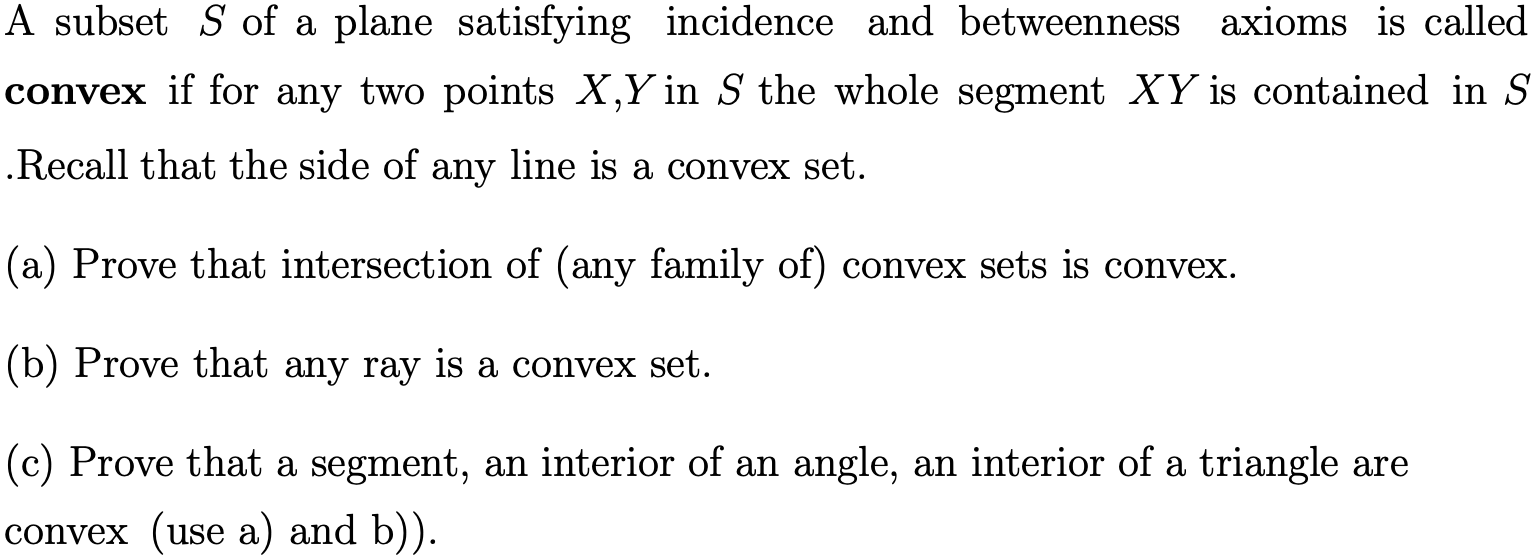# A subset S of a plane satisfying incidence and betweennessaxioms is calledconvex if for any two points X,Y in S the whole segment XY is contained in SRecall that the side of any line is a convex set.(a) Prove that intersection of (any family of) convex sets is convex(b) Prove that any ray is a convex set.(c) Prove that a segment, an interior of an angle, an interior of a triangle are(use a) and b))convex

Question
59 viewshelp_outlineImage TranscriptioncloseA subset S of a plane satisfying incidence and betweenness axioms is called convex if for any two points X,Y in S the whole segment XY is contained in S Recall that the side of any line is a convex set. (a) Prove that intersection of (any family of) convex sets is convex (b) Prove that any ray is a convex set. (c) Prove that a segment, an interior of an angle, an interior of a triangle are (use a) and b)) convex fullscreen
check_circle

Step 1

(a)

To prove: - Intersection of convex set is convex.

Let us take two convex set (let A and B) and C=A∩B

From the definition convex set, a subset S of a plane satisfying incidence and betweenness axioms is called convex if for any two points a, b in S the whole segment ab is contained in S.

Let x and y be two points in C then the segment xy belongs to both the set that is, in set A and set B. It is already known that both the set A and B are convex.

Hence segment formed by every pair of point in the set C is contained in the set C. Hence, we conclude that the set is convex.

Similarly, for the family of convex sets, the line segment formed by any two points in the intersection of the sets belongs to every convex set of the family which implies that the intersection of (any family of) convex sets is convex.

An example of the intersection of two circular disks is shown in the figure: -

In this figure, segment formed by any two points in C is contained in C.

Step 2

(b)

To prove: Any ray is convex set.

Let us consider a ray say AB and let us take any two arbitrary points C and D on the ray AB.

The line segment CD completely lies on the ray AB and from the definition of convex set, a subset S of a plane satisfying incidence and betweenness axioms ...

### Want to see the full answer?

See Solution

#### Want to see this answer and more?

Solutions are written by subject experts who are available 24/7. Questions are typically answered within 1 hour.*

See Solution
*Response times may vary by subject and question.
Tagged in

### Math